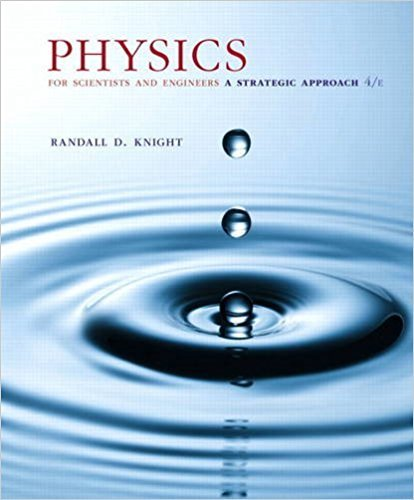×
×

# How are graphs used in kinematics?ISBN: 9780134081496 191

## Solution for problem 2.2 Chapter 2

Physics for Scientists and Engineers: A Strategic Approach, Standard Edition (Chs 1-36) | 4th Edition

• Textbook Solutions
• 2901 Step-by-step solutions solved by professors and subject experts
• Get 24/7 help from StudySoup virtual teaching assistantsPhysics for Scientists and Engineers: A Strategic Approach, Standard Edition (Chs 1-36) | 4th Edition

4 5 0 339 Reviews
22
3
Problem 2.2

How are graphs used in kinematics?

Step-by-Step Solution:
Step 1 of 3

Lectures 1 2 and 3 Average Speed & Average Velocity vavgdistancetime=dt *speed is a scalar (magnitude with no direction vavgdisplacementtime= rt * velocity is a vector (has direction) → UNITS: ms vavg and r point in direction of motion vx= xf-x0t → xf=x0+t(vx) *with constant velocity  Negative slope = negative...

Step 2 of 3

Step 3 of 3

##### ISBN: 9780134081496

Physics for Scientists and Engineers: A Strategic Approach, Standard Edition (Chs 1-36) was written by and is associated to the ISBN: 9780134081496. This textbook survival guide was created for the textbook: Physics for Scientists and Engineers: A Strategic Approach, Standard Edition (Chs 1-36), edition: 4. The answer to “How are graphs used in kinematics?” is broken down into a number of easy to follow steps, and 6 words. This full solution covers the following key subjects: . This expansive textbook survival guide covers 42 chapters, and 4463 solutions. Since the solution to 2.2 from 2 chapter was answered, more than 241 students have viewed the full step-by-step answer. The full step-by-step solution to problem: 2.2 from chapter: 2 was answered by , our top Physics solution expert on 12/28/17, 08:06PM.

Unlock Textbook Solution